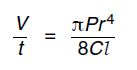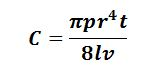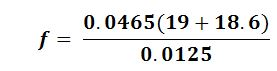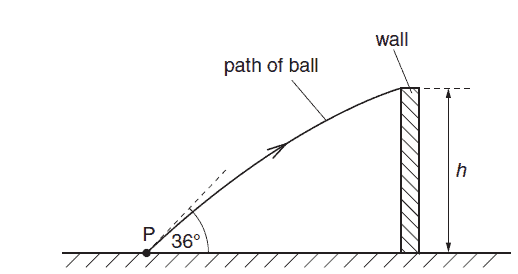Physic Tutorials

# How to solve questions on Measurement and Kinematics for Cambridge A level

The two questions are cambridge may/june 2012 p21

Q1

The volume V of liquid that flows through a pipe in time t is given by the equationwhere P is the pressure difference between the ends of the pipe of radius r and length l.
The constant C depends on the frictional effects of the liquid.
Determine the base units of C.

solution

The base unit of pressure isthe base unit for radius = m

the base unit for length = m

volume = m^3

t = s

the first thing to do is to make C the subject of the equationSubstitute all the base unitsQ2

A ball is thrown vertically down towards the ground with an initial velocity of 4.23 m s–1. The
ball falls for a time of 1.51 s before hitting the ground. Air resistance is negligible.
(a) (i) Show that the downwards velocity of the ball when it hits the ground is 19.0 m s–1.
(ii) Calculate, to three significant figures, the distance the ball falls to the ground.

(b) The ball makes contact with the ground for 12.5 ms and rebounds with an upwards
velocity of 18.6 m s–1. The mass of the ball is 46.5 g.
(i) Calculate the average force acting on the ball on impact with the ground

Solution

v = u + at

u = 4.23 ms-1

t = 1.51 s

a = 9.81

v = 4.23 + 1.51 *9.81

v = 19.04 ms-1

iis = 17.57 m

bF = 140 N

A ball of mass 400 g is thrown with an initial velocity of 30.0 m s–1 at an angle of 45.0° to the
horizontal, as shown in fig belowAir resistance is negligible. The ball reaches a maximum height H after a time of 2.16 s.
(i) Calculate
1. the initial kinetic energy of the ball,
2. the maximum height H of the ball

cambridge past que may / jun 2014 p22 q4

Solution

Ek = 1/2 m v2

m = 400g = 0.4kg

v = 30ms-1

Ek = 1/ 2 * 0.4 * 30 ^2 = 180 J

To calculate the maximum

v2=u2– 2as

v = 0

u is the vertical component of the velocity = usinθ = 30 sin 45 = 21.21 ms-1

s = 21.21^2 / 2* 9.81 = 22.94m

2.

A ball is thrown from a point P, which is at ground level, as illustrated in figure belowThe initial velocity of the ball is 12.4 m s–1 at an angle of 36° to the horizontal.
The ball just passes over a wall of height h. The ball reaches the wall 0.17 s after it has been
thrown.
Assuming air resistance to be negligible, calculate
(i) the horizontal distance of point P from the wall,
(ii) the height h of the wall.

cambridge past que oct / nov 2010 p22 que 2

Solution

the horizontal distance is

v = d/t

v = ucosθ = 12.4 cos 36 = 10.03 ms-1

d = vt = 10.03 * 0.17 = 1.7 m

the heignt h

s = ut – 1/2 a t2

the u here is the vertical component of the initial velocity usinθ = 12.4 sin 36 = 7.29 ms-1

s = 7.29 * 0.17 – 1/2 * 9.81 * 0.17^2 = 1.24 – 0.14 = 1.1 m

3.

An object is projected from a height of 80m above the ground with a velocity of 40ms-1 at an angle of 30o to the horizontal. The time of flight is
A. 16s      B. 10s       C. 8s        D. 4s
[g = 10ms-2]

Solution

T = 2usinθ / g

T  = 2 * 40 * sin 30 / 10

T = 40 / 10

T = 4 s

### Bolarinwa Olajire

A lecturer, Educationist, PhD student at FUNAAB, and a Blogger.